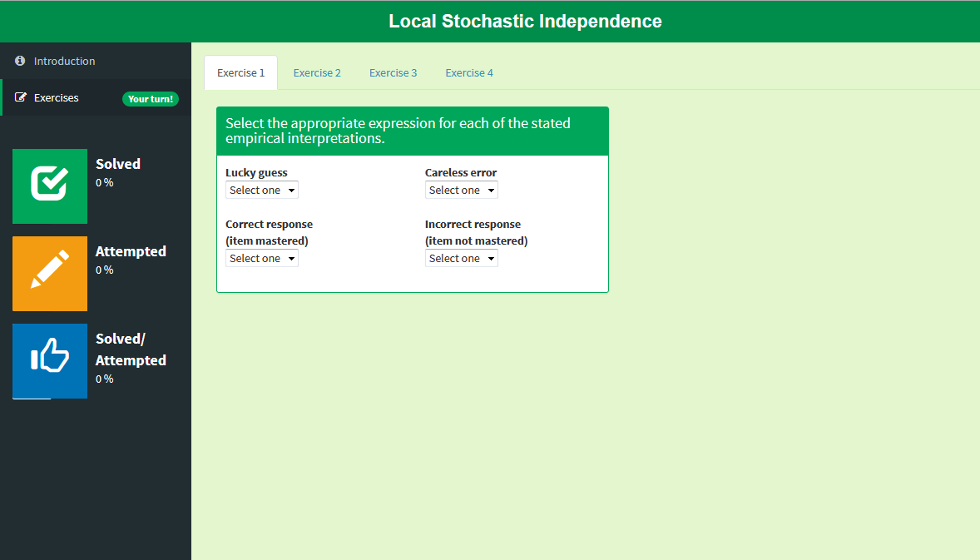This app exemplifies the local independence assumption of the basic local independence model (BLIM). This assumption is used to calculate the probability P(R|K) of a response pattern R given the knowledge state K. First the user learns the empirical interpretation of the model parameters. Next, an example with five items demonstrates the difference between correct responses and mastered items. After assigning to each item its correct interpretation, the corresponding part of the formula for P(R|K) is displayed. Finally, the app illustrates how P(R|K) changes with different parameter values.